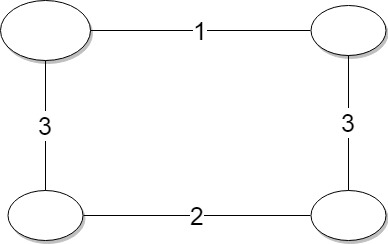Feb, Problem 2 | Fenced In

### Fenced In

##### Problem Statement

The large field is a rectangle with corner points at (0,0) and (A,B). FJ builds n vertical fences and m horizontal fences. Each vertical fence crosses through each horizontal fence, subdividing the large field into a total of (n+1)(m+1) regions. Unfortunately, FJ completely forgot to build gates into his fences, making it impossible for cows to leave their enclosing region and travel around the entire field! He wants to remedy this situation by removing pieces of some of his fences to allow cows to travel between adjacent regions. He wants to select certain pairs of adjacent regions and remove the entire length of fence separating them; afterwards, he wants cows to be able to wander through these openings so they can travel anywhere in his larger field.

For example,

FJ might take a fence pattern looking like this: +—+—+ |⠀⠀⠀|⠀⠀ | +—+—+ |⠀⠀⠀|⠀⠀ | |⠀⠀⠀|⠀⠀ | +—+—+ and open it up like so: +—+—+ |⠀⠀⠀ ⠀⠀ | +—+⠀⠀+ |⠀⠀⠀ ⠀⠀ | |⠀⠀⠀ ⠀⠀ | +—+—+

#### MST

The key insight is that we can represent this problem as a Minimum Spanning Tree (MST). We represent each individual grid as a node on the MST. Edges between nodes are the fences that FJ can remove. Specifically, the edge length is the length of the fence.

As an example, we can represent the above diagram as thus.#### Kruskal’s MST Algo

If we try to solve this the naive way, we get timed out. Traditional MST algorithms run in O(N log N). Because we actually have N^2 regions, this means our runtime is O(N^2 log N).

Note that for every horizontal edge, there are a total of n other edges with the same length. Similar logic applies for vertical edges, with a total of m instead.

Consider the edge with shortest length. By Kruskal’s, we need to add as many of these edges as possible, as long as we keep our tree. The exact number of times we need to add the edge can be calculated by subtracting the number of edges in the opposite orientation already added from the max amount.

``````edgesToAdd = (m - verticalLines) if currentEdge.horizontal else (n - horizontalLines)
``````

#### Code

My solution can be found here

Written by Robert Chen

Notice any mistakes? Please email us at [email protected] so that we can fix any inaccuracies.